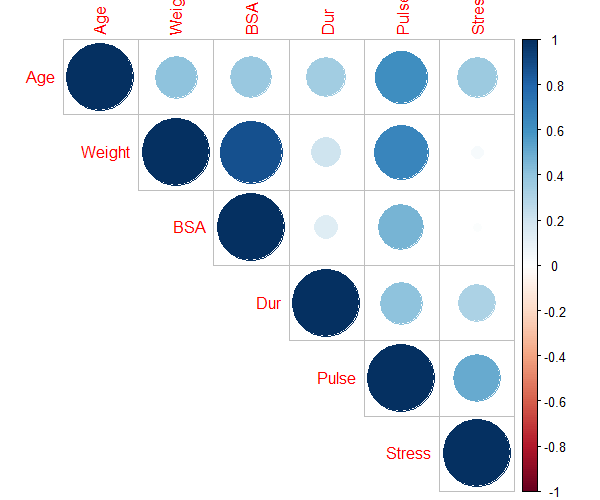# How to Calculate and Interpret Variance Inflation Factor (VIF) in R

## What is Variance Inflation Factor (VIF)?

Variance inflation factor (VIF) is a most commonly used metric for measuring the degree of multicollinearity in the regression model.

Multicollinearity refers to the existence of a high correlation between two or more independent variables in the regression model.

Multicollinearity is problematic in the regression as it leads to a biased and unstable estimation of regression coefficients, increases the variance and standard error of regression coefficients, and decreases the statistical power.

## How to interpret VIF?

The VIF value ranges from +1 to the positive infinity. The VIF value of 1 indicates a complete absence of multicollinearity.

The following VIF ranges are mostly used for assessing the moderate to the severity of multicollinearity,Note: There is no universally accepted range for VIF values for multicollinearity detection. It is advisable to have VIF < 2.

## How to calculate VIF in R?

We will use the blood pressure example dataset for calculating the VIF in R. This dataset contains `Age`, `weight`, `BSA`, `Dur`, `pulse`, and `Stress` predictors (independent variables) and `BP` as response variable (dependent variable).

In this example, our goal is to calculate VIF and to check if there is multicollinearity exists within the six predictor variables.

``````# load dataset

# view first few rows
BP Age Weight  BSA  Dur Pulse Stress
1  105  47   85.4 1.75  5.1    63     33
2  115  49   94.2 2.10  3.8    70     14
3  116  49   95.3 1.98  8.2    72     10
4  117  50   94.7 2.01  5.8    73     99
5  112  51   89.4 1.89  7.0    72     95
6  121  48   99.5 2.25  9.3    71     10
``````

Fit the multiple regression model,

``````# fit the regression model
model <- lm(BP ~ Age + Weight + BSA + Dur + Pulse + Stress, data = df)

# get the F statistics and performance metrics
summary(model)\$fstatistic
value
560.641

summary(model)\$r.squared
0.9961

0.9943
``````

The higher F value suggests that there is a significant relationship between the predictor variables and the response variable (`BP`).

The higher adjusted R-Squared (0.9943) also suggests that the fitted model has better performance and explains most of the variation in the response variable that can be explained by predictor variables.

Now, we will calculate the VIF to check whether there is multicollinearity exists among the predictor variables.

In R, VIF can be calculated using the `vif()` function (from the `car` package).

``````# load package
library(car)

# calculate VIF for each predictor variable from fitted model
vif(model)

Age   Weight      BSA      Dur    Pulse   Stress
1.762807 8.417035 5.328751 1.237309 4.413575 1.834845
``````

The VIF values for `weight`, `BSA`, and `pulse` are high (VIF > 2) and suggest that these variables are highly correlated with some predictor variables in the model. It means that there is multicollinearity exists among the predictor variables.

## How to remove variables causing multicollinearity?

To check which variables are highly correlated and causing the multicollinearity, you can perform the pairwise correlation analysis for predictor variables.

You can use the `cor()` function to perform the pairwise correlation analysis using a data frame.

``````# pairwise correlation analysis
# exclude response variable (BP)
cor(df[ , -1])

Age     Weight        BSA       Dur     Pulse     Stress
Age    1.0000000 0.40734926 0.37845460 0.3437921 0.6187643 0.36822369
Weight 0.4073493 1.00000000 0.87530481 0.2006496 0.6593399 0.03435475
BSA    0.3784546 0.87530481 1.00000000 0.1305400 0.4648188 0.01844634
Dur    0.3437921 0.20064959 0.13054001 1.0000000 0.4015144 0.31163982
Pulse  0.6187643 0.65933987 0.46481881 0.4015144 1.0000000 0.50631008
Stress 0.3682237 0.03435475 0.01844634 0.3116398 0.5063101 1.00000000
``````

Visualize the pairwise correlation using `corplot()` function from `corrplot` R package,

``````# load package
library(corrplot)

# visualize pairwise correlation
corrplot(cor(df[ , -1]), type = "upper")
``````The pairwise correlation suggests,

• `Weight` is highly correlated with `BSA` (r > 0.8) and `Pulse` (r > 0.6)
• `Pulse` is highly correlated with `Age` (r > 0.6)

Based on VIF and pairwise correlation analysis, we can remove the `BSA` and `Pulse` variables to remove the potential multicollinearity among the predictor variables.

Now, re-fit the regression model with the new dataset (after removing `BSA` and `Pulse` variables) and check if there is multicollinearity exists among the predictor variables.

As per pairwise correlation analysis, `Weight` is highly correlated with `BSA` (r > 0.8) and `Pulse` (r > 0.6). We will drop the `BSA` and `Pulse` variables from regression model.

``````# get new dataset
df_new <- df[, c("BP", "Age","Weight", "Dur", "Stress")]

# fit the regression model
model <- lm(BP ~ Age + Weight +  Dur + Stress, data = df_new)

# calculate VIF
vif(model)

Age   Weight      Dur   Stress
1.468245 1.234653 1.200060 1.241117
``````

As you can see, in the updated regression model, there is no strong multicollinearity among the predictor variables.

Hence, these four variables could be used as predictor variables in regression analysis. This process of selection of appropriate variables is also known as feature selection.

How to fix multicollinearity?

1. Increase the sample size
2. Remove variables causing multicollinearity
3. Combine the highly correlated predictor variables

## Enhance your skills with statistical courses using R

1. Vatcheva KP, Lee M, McCormick JB, Rahbar MH. Multicollinearity in regression analyses conducted in epidemiologic studies.
Epidemiology (Sunnyvale, Calif.). 2016 Apr;6(2).
2. Daoud JI. Multicollinearity and regression analysis. InJournal of Physics: Conference Series 2017 Dec 1 (Vol. 949, No. 1, p. 012009). IOP Publishing.
3. Detecting Multicollinearity Using Variance Inflation Factors